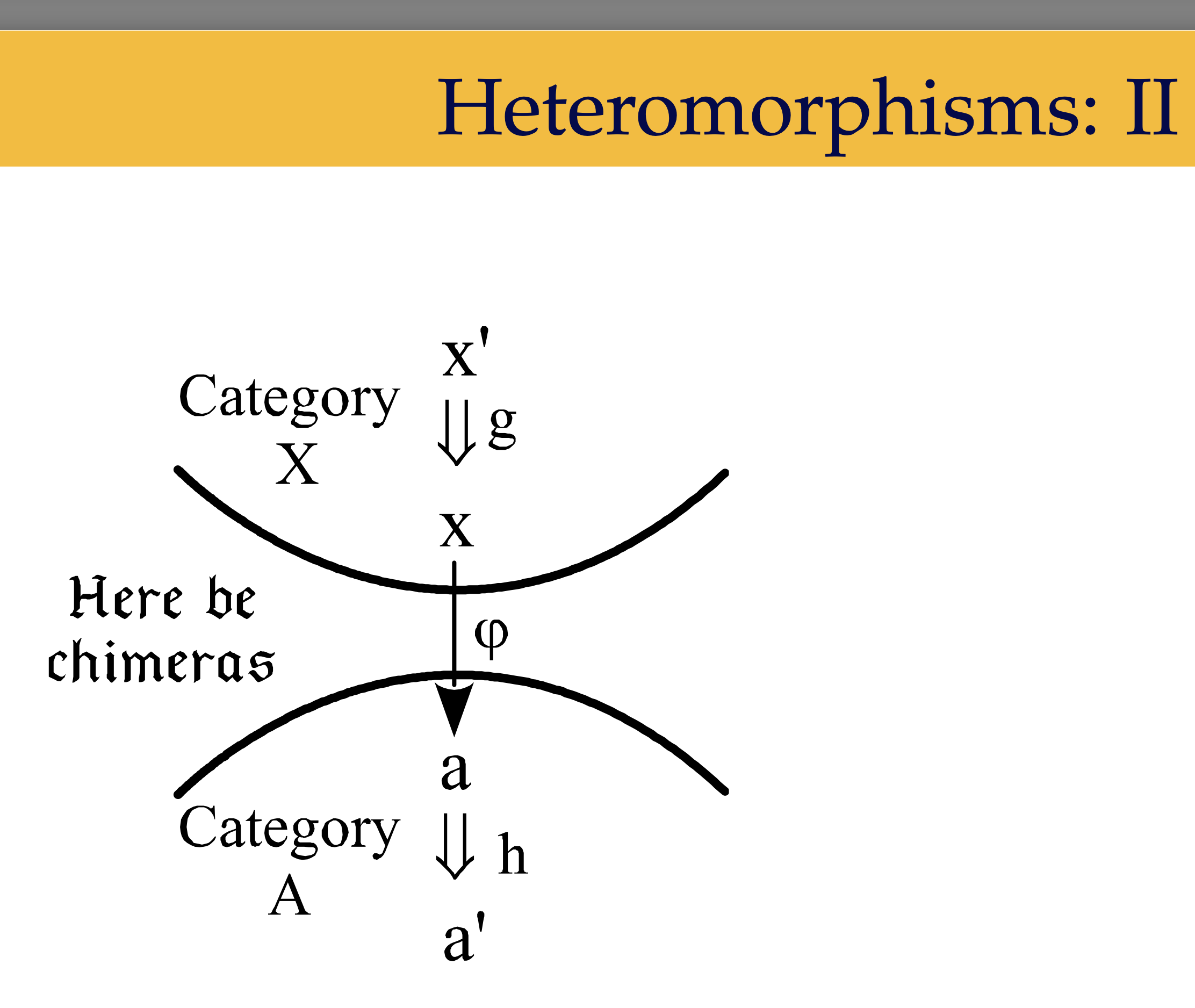# Profunctors generalize relations

⇐ Notes archive

(This is an entry in my technical notebook. There will likely be typos, mistakes, or wider logical leaps — the intent here is to “let others look over my shoulder while I figure things out.”)

An aside before the note, Day One reminded me that I started studying category theory, in earnest, almost a year ago today.

Despite being a hobby, I’m pretty proud of how far I’ve come (I struggle with saying that aloud, since I’m the type to keep pushing forward and not really look around along the way).

I’m

Richard Guy put how I’ve felt as of late succinctly.

…and I love anybody who can [do mathematics] well, so I just like to hang on and try to copy them as best I can, even though I’m not really in their league.

(I’m not sure if anyone reads these entries hah (quite literally, I removed analytics on the site a few years ago). If so, pardon the moment to reflect.)

I was revisiting profunctors yesterday and Bartosz mentioned an intuition in lecture III.6.1 (timestamped) that made their motivation click.

You can think of a profunctor as [generalizing] a relation between objects.

Huh, okay. Let’s take a step back and recap what a relation is in plain ol’ set theory and follow the intuition.

A binary relation over two sets $X$ and $Y$ is a subset of their Cartesian product, $X \times Y$. That is, a set of ordered pairs indicating which $X$s are related to specific $Y$s.

And now to generalize.

First, let’s swap out the sets for categories $C$ and $D$.

$C \times D$. Okay, the product category. Since $C$ and $D$ are possibly distinct categories, we can’t directly consider morphisms between them. But, we can in their product category—morphisms between objects $(c, d)$ and $(c^\prime, d^\prime)$ are those in the form $(f, g)$ with $f: c \rightarrow c^\prime$ and $g: d \rightarrow d^\prime$. So, in a sense, the collection of relationships between $c$ and $d$ is $\textrm{Hom}((c, d), (c^\prime, d^\prime))$.

That hom-set is, well, a set (assuming we’re working with small categories)! What if we tried to create a functor from $C \times D \rightarrow \mathbf{Set}$ defined by $(c, d) \mapsto \ldots$

Wait. $c$ and $d$ come from different categories and hom-sets only work in a single category. I read around to reconcile this and stumbled upon heteromorphisms. “Morphisms” between two objects from different categories that use a bridging functor to then construct a hom-set. I got lost trying to read further, and with warning from slide 3/41 of David Ellerman’s presentation on the topic.So, let’s assume $C = D$ and carry on (I’ll understand that presentation someday).

Okay, let’s map $c, d$ (both objects in $C$) to $\textrm{Hom}(c, d)$. And for morphisms, we need to map some $(f, g)$ for $f: c \rightarrow c^\prime$ and $g: d \rightarrow d^\prime$ to a function between $\textrm{Hom}(c, d)$ and $\textrm{Hom}(c^\prime, d^\prime)$. Let’s pluck out a morphism, say $h$ from $\textrm{Hom}(c, d)$.

We have $f, g, h$ in hand and need to construct a morphism from $c^\prime$ to $d^\prime$. There’s…no way to do this. None of our morphisms map from $c^\prime$.

That’s where the contravariance in the profunctor construction comes from when folks write $C^{\textrm{op}} \times C \rightarrow \mathbf{Set}$ (or, in the general case $C^{\textrm{op}} \times D \rightarrow \mathbf{Set}$). Taking the dual in the first component of the product category flips $f$ and now lets us get from $c^\prime$ to $d^\prime$ by way of $g \circ h \circ f$.

It’s okay if you need to walk around the park with that composition before it makes sense. I certainly needed to and it demystified the rogue dimap’s I’d see in Preludes.

But, let’s take stock on how this generalizes relations. In the same-category setting, we’re constructing a functor that maps two objects to the ways in which they’re related, their hom-sets. Since it’s a functor, we also need to consider mapping morphisms across the functor into functions between hom-sets and dimap (link to Haskell’s Prelude) does just that.